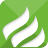40年專注研發生產集一體的電阻元器件供應鏈

## 聯系我們## 精密電阻阻值對照表

### 文章來源：插件電阻人氣：4589發表時間：2018-11-07 14:39:34【小中大】

眾所周知，精密電阻一般是指精度高(萬分之一以上)、溫漂低(10ppm以下)及長期穩定性(年變化率小于50ppm)的電阻，它相比于金屬膜電阻、線繞電阻、金屬箔電阻等電阻來說要精密得多。而在精密電阻的使用過程中，與阻值的關系往往密不可分。

精密電阻的阻值，在一般情況下是由兩位數字加一位字母表示(也有一些是由4位數字組成)，如：12D，28D……等。但是，不少的人即使是了解阻值的計算，仍然會感覺比較難以計算。在這時，精密電阻阻值對照表的重要性就顯示出來了。

精密電阻阻值對照表：其中表格的最后兩行的A，B，C，D，E，F，X，Y是數量級代表符號，用字母來表示，則是：

A=10^0=1

B=10^1=10

C=10^2=100

D=10^3=1000

E=10^4=10000

F=10^5=100000

X=R=10^(-1)=1/10

Y=S=10^(-2)=1/100

舉個例子：

24A所表示的阻值為：174×100=174×1=174?R(歐姆)?

24Y所表示的阻值為：174×10-2=1.74?R(歐姆)

24X：17.4(歐姆)

24B：1.74K(歐姆)

24C：17.4K?(歐姆)

24D所表示的阻值為：174×103?=174×1000=174?KΩ(千歐)

以上所介紹的內容，就是精密電阻阻值對照表，相信通過表格能讓大家對于精密電阻阻值能有一個更清晰的了解。當然，精密電阻的種類還有很多，除了以上介紹外，可能還有其他阻值的精密電阻，冠發小編在這里就不再一一介紹了。

0.1061s0.1099s